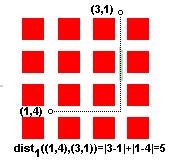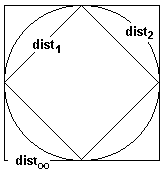Things that are distant though near: Brothers, sisters, and other members of a family that do not love each other. Things that are near though distant: Paradise. Sei Shonagon (born circa 965), The Pillow Book, translated by Ivan Morris, Penguin Classics, 1967.

Just think of this. One thousand years ago a person of a completely different culture conceived a notion that would probably be endorsed today by nearly every one. Physical proximity is unfortunately often not a reflection of moral or intellectual proximity. Are we any different from those born in the distant past of one thousand years ago? You do feel quite close and involved when they share their thoughts and emotions with you, do not you? Well, by now you are probably near wondering whether a discussion on human nature is a sensible topic for a mathematical page. Have I strayed too far from the topic I want to discuss?I plan to discuss the mathematical notion of distance. You may or may not remember that the most customary notion of the distance is somehow related to the Pythagorean Theorem. But having read the introduction you won't be surprised to learn that mathematicians study other distances as well. However, once they realized what is actually important about the notion of distance, they managed to distill its three most significant properties. These are the axioms of distance. A function dist(A,B) of two variables defined on a set (Metric Space) M is called a distance (between A and B), provided

1. 2. dist(A, B)) ≥ 0 and dist(A, B) = 0 if and only if A = B. (Positiveness) dist(A, B) = dist(B, A) (Symmetry) dist(A, B) + dist(B, C) ≥ dist(A, C) (the Triangle Inequality)Let's consider several examples.1. M = R, the set of all real numbers. dist(a, b) = |a - b|, where |x| is the absolute value of x.
2. M = R2, the plane, i.e. the set of all points with 2 coordinates (x, y). dist1((x1, y1),(x2, y2)) = |x2 - x1| + |y2 - y1|. This is known as the city-block or taxicab distance. Why? Because it's not always one can cut corners. Sometimes you just do the best you can. To get from the corner of First Avenue and 4th Street to the corner of the Third Avenue and 1st Street you have to pass 5 blocks regardless of a particular itinerary you choose. (City-block distance helps solve one of Sam Loyd's puzzles.)
3. M = R2. dist2((x1, y1),(x2, y2)) = ((x2 - x1)2 + (y2 - y1)2)1/2. This is the well known Euclidean distance.
4. M = R2. dist((x1, y1),(x2, y2)) = max{|x2 - x1|, |y2 - y1|}.
5. M = S2. A circle (of radius 1) in the plane. The distance is defined as before, for the whole R2.
6. M = S2. Points on a circle are identified with the corresponding central angle. The distance dista is the angular distance: the angle is assumed to change in the interval [0, π], i.e. to be the smallest (non-negative) central angle corresponding to the two points.
7. M = R3. dist1 and dist2 have a natural extension into a 3- or, for that matter, higher dimensional spaces.
8. M = C[0, 1] - the space of all functions continuous on the interval [0,1]. dist(f, g) = max|g(x) - f(x)|, x∈[0, 1].
9. Hausdorff distance gives us a measure of nearness between sets.
10. Bounded Distance
11. Distance between stringsDistance, the metric function, is the mathematical abstraction of measurement. Given a distance function we may answer several often useful questions that have a very common meaning. Is point B farther away from point A than point C? Are points B and C equidistant from A? What is the locus of points whose distance to A is exactly R? Mathematically the answer to the last question is {X: dist(X, A) = R} - the set of all points X such that dist(X, A) = R. This is a generalization of a circle (in R2) and a sphere (in R3). It's customary to call this set a sphere SR(A) = {X: dist(X, A) = R} with radius R centered at A. It's instructive to consider some examples.

Consider the plane with the distance defined by dist1. What is S1(0, 0)?In other words, how does the set S1(0, 0) = {(x, y): dist1((x, y), (0, 0)) = 1} look like?. From the definition, S1(0, 0) = {(x, y): |x| + |y| = 1}. The set is the union of four straight line segments: x + y = 1 in the first quadrant, y - x = 1 in the second, -y - x = 1 in the third, and -y + x = 1 in the fourth. It's a square with vertices on the axes.

For dist in the plane the sphere S(0, 0) is also a square but now its sides are parallel to the axes.

Observe that for a pair of points X and Y

 dist∞(X, Y) ≤ dist2(X, Y) ≤ dist1(X, Y).

However, the unit spheres corresponding to the three distances are included in the reverse order. Why?Thus it's clear that once several distance functions have been defined on the same set the expression "the distance between two points" becomes ambiguous. However, as is suggested by the spheres diagram above, points in R2 "close" in one of the distances dist, dist1, dist2 will be close in the others. More accurately, the following is true:

### Proposition

Let a sequence of plane points A, A1, A2, A3, ... be such that disti(A, Ak)->0 as k goes to infinity for i being one of ∞, 1, or 2. Then the same is true for the remaining values of i.

### Proof

The Proposition follows from the following series of inequalities:

 dist∞(X,Y) ≤ dist2(X, Y) ≤ dist1(X, Y) ≤ 2dist∞(X, Y)Now it's time to fulfill the implicit promise made at the beginning of the page. Consider what's known as the Stereographic projection. The name derives from a configuration in R3 but I'll use its 2-dimensional analog. A circle stands on a straight line. Through its North pole O and the points of the horizontal axis draw straight lines and note the points of intersection with the circle. So that points A,B,C from the circle correspond to the points A',B', and C' on the x-axis. This is a 1-1 correspondence between points of a straight line and points on a circle without its North pole. Let's define the distance on the latter set, S2-{North pole}, as dists(X, Y) = dist2(X', Y'). Then it's clear that the points close in the angular dista may be far apart in the distance dists. To see this pick up points near to but on opposite sides of the pole.

The stereographic projection works both ways. So that the formula distr(X', Y') = dista(X, Y) defines a new distance function on R1. It's easy to see that there are points on the axis distant in, say, dist2 but close in distr.### Taxicab/City-block Distance.### Stereographic Projection